Most Affordable JEE | NEET | 8,9,10 Preparation by Kota's Top IITian Doctor Faculties

# NCERT Solutions for Class 12 Chemistry Chapter 1 The Solid State PDF - eSaral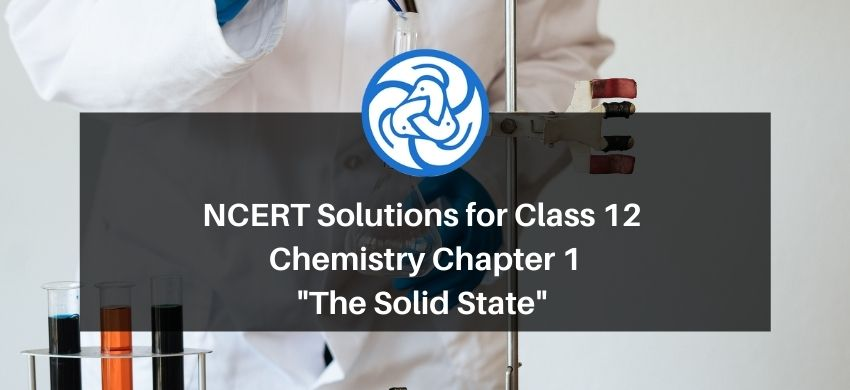`

# NCERT Solutions for Class 12 Chemistry Chapter 1 The Solid State PDF

Hey, are you a class 12 student and looking for ways to download NCERT Solutions for Class 12 Chemistry Chapter 1 The Solid State PDF? If yes. Then read this post till the end.

In this article, we have listed NCERT Solutions for Class 12 Chemistry Chapter 1 The Solid State in PDF that are prepared by Kota’s top IITian Faculties by keeping Simplicity in mind.

If you want to learn and understand class 12 Chemistry Chapter 1 "The Solid State" in an easy way then you can use these solutions PDF.

NCERT Solutions helps students to Practice important concepts of subjects easily. Class 12 Chemistry solutions provide detailed explanations of all the NCERT questions that students can use to clear their doubts instantly.

If you want to score high in your class 12 Chemistry Exam then it is very important for you to have a good knowledge of all the important topics, so to learn and practice those topics you can use eSaral NCERT Solutions.

So, without wasting more time Let’s start.

### Download NCERT Solutions for Class 12 Chemistry Chapter 1 The Solid State PDF

Question 1. Why are solids rigid?

Solution. The particles of solids are close to each other, and they are tightly bonded, having high intermolecular forces of attraction between molecules. So they cannot move from their positions as the interparticle space is very less. However, they can only oscillate about their mean positions.

Question 2. Why do solids have a definite volume?

Solution: Intermolecular forces of attraction that are present in solids are very strong, and constituent particles are very close and are not free to move around. Thus, solids possess rigidity and have a definite volume.

Question 3. Classify the following as amorphous or crystalline solids: Polyurethane, naphthalene, benzoic acid, Teflon, potassium nitrate, cellophane, polyvinyl chloride, fibreglass, copper.

Solution: Amorphous solids are Polyurethane, Teflon, cellophane, polyvinyl chloride, fibreglass.

The atoms and molecules are not in a definite lattice pattern. In these solids, the constituent particles (atoms, ions or molecules) have short range order of arrangement.

Crystalline solids are Naphthalene, benzoic acid, potassium nitrate, copper are crystalline solids because constituents (such as atoms, molecules, or ions) are arranged in a highly ordered microscopic structure, forming a crystal lattice that extends in all directions.

Question 4. Refractive index of a solid is observed to have the same value along all directions. Comment on the nature of this solid. Would it show the cleavage property?

Solution. As the solid has the same value of the refractive index in all directions, this means that it is isotropic and hence amorphous. When an amorphous solid is cut, it cuts into two pieces with irregular surfaces. These solids do not show cleavage.

Question.5 Why is glass considered a super cooled liquid?

Solution. Similar to liquids, glass has a tendency to flow, though very slowly. Therefore, glass is considered as a super cooled liquid. This is the reason that glass windows and doors are slightly thicker at the bottom than at the top.

Question 6. Classify the following solids in different categories based on the nature of intermolecular forces operating in them: Potassium sulphate, tin, benzene, urea, ammonia, water, zinc sulphide, graphite, rubidium, argon, silicon carbide.

Solution. Potassium sulphate $\rightarrow$ Ionic solid

Potassium sulphate has $2 \mathrm{~K}^{+}$and $\mathrm{SO}_{4}^{-}$. It consists of positively charged cations and negatively charged anion. When an ionic solid is dissolved in water, the cations and anions are separated and become free to move about in the water allowing the solution to conduct electrical current.

Tin → Metallic solid

Metallic solids are solids composed of metal atoms that are held together by metallic bonds. These bonds are like huge molecular orbitals that span across the whole solid. This means the electrons in metallic solids are delocalized.

Benzene → Molecular (non-polar) solid

Non-polar molecular solid: In these solids, the atoms or molecules are held by weak dispersion forces or London forces. These solids are soft and non-conductors of electricity

Urea → Polar molecular solid

Polar molecular solids: In these types of solids, the geometry is in such a way that one side has a negative charge, and the other has a positive charge. The force between them is a dipole-dipole force of attraction. The melting and boiling points of these solids are higher than non-polar molecular solids but still relatively low.

Ammonia → Polar molecular solid

Polar Molecular Solids: In these types of solids, the geometry is in such a way that one side has a negative charge, and the other has a positive charge. The force between them is a dipole-dipole force of attraction. The melting and boiling points of these solids are higher than non-polar molecular solids but still relatively low.

Water → Hydrogen bonded molecular solid

Hydrogen-bonded molecular solids contain polar covalent bonds between $\mathrm{H}$ and F, 0 or $\mathrm{N}$ atoms. Strong hydrogen bonding binds molecules of such solids like $\mathrm{H}_{2} \mathrm{O}$ (ice).

They are non-conductors of electricity and are volatile liquids or soft solids under room temperature and pressure.

Zinc sulphide → Ionic solid

Zinc sulphide has positively charged cation $\left(\mathrm{Zn}^{+2}\right)$ and negatively charged anion $\left(\mathrm{S}^{2-}\right) .$ When ionic solid is dissolved in water, the cations and the anions get separated and become free to move in the water allowing the solution to conduct electrical current.

Metallic solids are solids composed of metal atoms that are held together by metallic bonds. These bonds are like huge molecular orbitals that span across the whole solid. This means the electrons in metallic solids are delocalized.

Graphite→ Covalent or network solid

A network solid or covalent network solid is a type of solid in which the atoms present are bonded by covalent bonds in a continuous network that extends throughout the material. In a network solid there are no individual molecules, and the entire crystal or amorphous solid may be considered a macromolecule.

Rubidium→ Metallic solid

Argon → Non-polar molecular solid

Non-polar molecular solids: In these solids, the atoms or molecules are held together by weak dispersion forces or London forces. These solids are soft and non- conductors of electricity

Silicon carbide → Covalent or network solid

Silicon carbide makes a network structure by covalent bonds.

Question 7. Solid A is a very hard electrical insulator in solid as well as in molten state and melts at extremely high temperature. What type of solid is it?

Solution: Solid A is hard and acts as an electrical insulator in molten as well as solid state. It can be either hydrogen-bonded molecular solid or covalent solid. Since it has a high melting point, it is a covalent or network solid. Covalent Network solid examples are diamond and quartz $\left(\mathrm{Si} \mathrm{O}_{2}\right)$.

Question 8. Ionic solids conduct electricity in the molten state but not in a solid state. Explain.

Solution: Ionic solids dissociate to give free ions, and electricity is conducted by ions in an aqueous or molten state. In the solid state, ions are held together by strong electrostatic forces and are not free to move. So, it is a bad conductor of electricity in the solid state.

Question 9. What type of solids are electrical conductors, malleable and ductile?

Solution: Metallic solids are solids composed of metal atoms that are held together by metallic bonds. These bonds are like huge molecular orbitals that span across the whole solid. This means the electrons in metallic solids are delocalized. So, they are a good conductor of electricity, and they are malleable and ductile.

Question 10. Give the significance of a ‘lattice point’.

Solution. The space lattice is strictly a geometrical concept and represents a threedimensional translational repetition of the centres of the units of pattern in the crystal. The unit of the pattern can be anything, (i) In metals or inert gases, each lattice point may represent the position of each atom. (ii) In a crystal of methane, each lattice point may represent the centre of a methane molecule. (iii) In ionic crystals, the lattice points may represent ions or ion-pairs

Question 11. Name the parameters that characterise a unit cell.

Solution. A unit cell is characterized by the following six parameters:

(i) The three edges (dimensions) a, b, and c.

(ii) Angles between the edges: $\alpha$ (between edges $\mathrm{b}$ and $\mathrm{c}), \beta$ (between edges a and $\mathrm{c}$ ), and $\gamma$ (between edges a and b).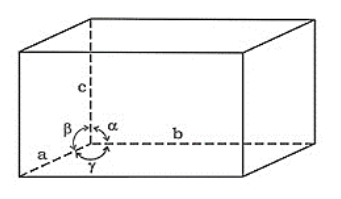Question 12. Distinguish between

(i) Hexagonal and monoclinic unit cells

(ii) Face-centred and end-centred unit cells.

Solution: (i) Hexagonal unit cell

For a hexagonal unit cell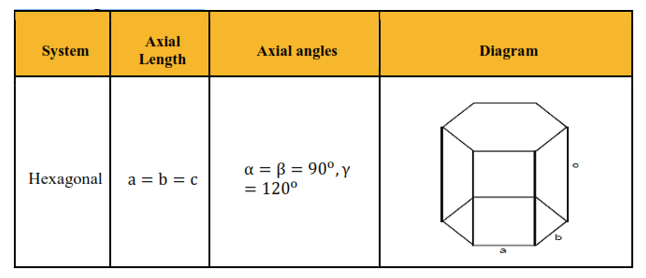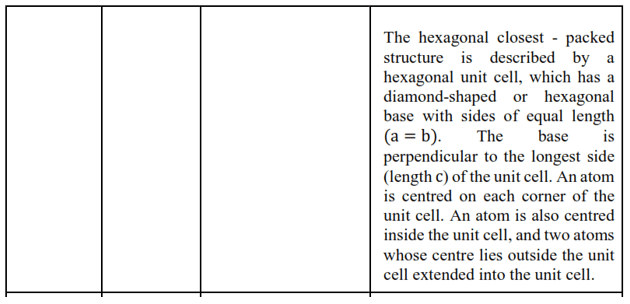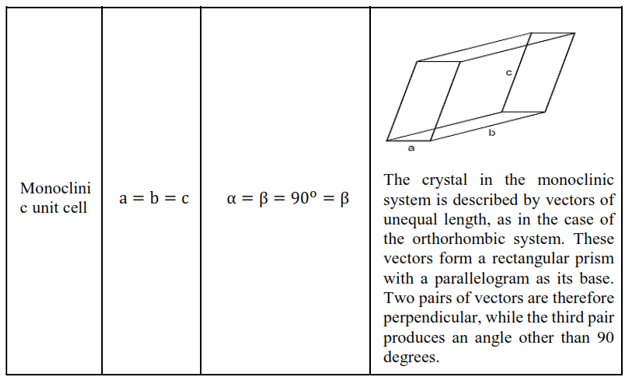(ii)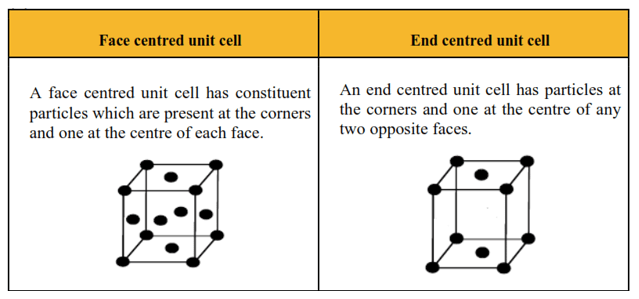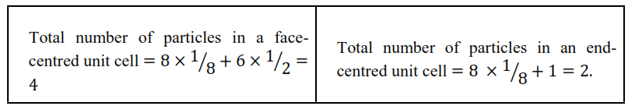Question 13. Explain how much portion of an atom located at (i) corner and (ii) body centre of a cubic unit cell is part of its neighbouring unit cell.

Solution: (i) An atom at the corner of a cubic unit cell is shared by eight adjacent unit cells.

Thus, $\frac{1}{8}$ th portion of the atom is shared by one unit cell.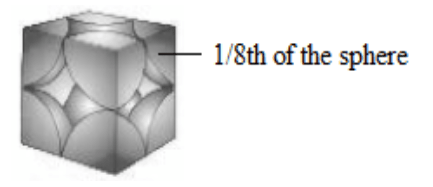(ii) An atom at the body centre of a cubic unit cell is not shared by its neighbouring unit cell. The atom is therefore only part of the unit cell in which it is present, i.e. its contribution to the unit cell is 1.

Question 14. What is the two-dimensional coordination number of a molecule in square closepacked layer?

Solution.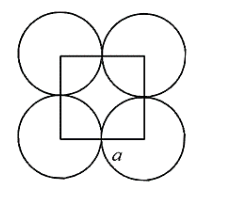A molecule is in contact with four of its neighbours in a two-dimensional square close-packed layer. Therefore, a molecule's two-dimensional coordination number in a close-packed square layer is 4 .

Question 15. A compound forms a hexagonal close-packed structure. What is the total number of voids in $0.5 \mathrm{~mol}$ of it? How many of these are tetrahedral voids?

Solution. Number of atoms in 1 mole of close-packed structure $=\mathrm{N}_{\mathrm{A}}=6.022 \times 10^{23}$

Number of atoms in $0.5$ moles of close-packed structure $=0.5 \times 6.022 \times 10^{23}=$ $3.011 \times 10^{23}$

Therefore, number of octahedral voids $=$ number of atoms per unit cell $=$ $3.011 \times 10^{23}$

And, number of tetrahedral voids $=$ twice the number of atoms per unit cell = $2 \times 3.011 \times 10^{23}=6.022 \times 10^{23}$

Therefore, total number of voids $=$ octahedral voids $+$ tetrahedral voids $=$ $3.011 \times 10^{23}+6.022 \times 10^{23}=9.033 \times 10^{23}$

Question 16. A compound is formed by two elements $\mathrm{M}$ and $\mathrm{N}$. The element $\mathrm{N}$ forms ccp and atoms of $\mathrm{M}$ occupy $1 / 3^{\mathrm{rd}}$ of tetrahedral voids. What is the formula of the compound?

Solution. Suppose the atoms of the elements N form the ccp lattice.

The amount of tetrahedral voids generated here is twice that of number of atoms of the element N, i. e. 2N

The Question states that the atoms of element M occupy one-third of the tetrahedral voids.

$\therefore$ The number of atoms of $\mathrm{M}$ is equal to $2 \mathrm{~N} \times 1 / 3=2 / 3^{\mathrm{rd}}$ of the number of atoms of $\mathrm{N}$.

Thus, the formula of the compound is $\mathrm{M}_{2 / 3} \mathrm{~N}=\mathrm{M}_{2} \mathrm{~N}_{3}$.

Question 17. Which of the following lattices has the highest packing efficiency.

(i) simple cubic

(ii) body-centred cubic and

(iii) hexagonal close-packed lattice?

Solution: The packing efficiency of hexagonal close-packing is

$=\frac{\text { Volume of the atoms }}{\text { Valume of unit cell }}=\frac{6 \times \frac{4}{3} \pi \mathrm{r}^{3}}{24 \sqrt{2} \mathrm{r}^{2}}$

$=\frac{\pi}{3 \sqrt{2}}=0.74=74 \%$

The packing efficiencies of simple cubic $=\frac{\text { volume occupid by atoms/unit cell }}{\text { volume of unit cell }}$

$=\frac{\frac{4}{3} \pi \mathrm{r}^{3}}{\mathrm{a}^{3}}=\frac{4}{3(2 \mathrm{r})^{3}} \pi \mathrm{r}^{3}=\frac{4 \pi \mathrm{r}^{3}}{3 \times 8 \mathrm{r}^{3}}=0.52(52 \%)$

The packing efficiencies of body-centred cubic lattices $=\frac{2 \times \frac{4}{3} \pi r^{3}}{4 \times 4 \times 4 \mathrm{r}^{3}} \sqrt{3} \times \sqrt{3} \times \sqrt{3}=$

$\frac{\pi \sqrt{3}}{8} \cong 0.68(68 \%)$

Question 18. An element with molar mass $2.7 \times 10^{-2} \mathrm{~kg} \mathrm{~mol}^{-1}$ forms a cubic unit cell with edge length $405 \mathrm{pm}$. If its density is $2.7 \times 10^{3} \mathrm{~kg} \mathrm{~m}^{-3}$, what is the nature of the cubic unit cell?

Solution. Given:- Density of the element $(\mathrm{d})=2.7 \times 10^{3} \mathrm{~g} \mathrm{~m}^{-3}$

Molar mass $(\mathrm{M})=2.7 \times 10^{-2} \mathrm{~kg} \mathrm{~mol}^{-2}$

Edge length $(\mathrm{a})=405 \mathrm{pm}=405 \times 10^{-12}$ meter $=4.05 \times 10^{-10}$ meter

It is known that, Avogadro number $\left(\mathrm{N}_{\mathrm{A}}\right)=6.022 \times 10^{23} \mathrm{~mol}^{-1}$

To find : The nature of the cubic unit cell

For this, we should know the number of atoms per unit cell (N)

Using the formula,

$\mathrm{d}=\frac{\mathrm{NM}}{\mathrm{a}^{3} \cdot \mathrm{N}_{\mathrm{A}}}$

$\mathrm{N}=\left(\mathrm{d} \cdot \mathrm{a}^{3} \cdot \mathrm{N}_{\mathrm{A}}\right) / \mathrm{M}$

$=2.7 \times 10^{3} \mathrm{kgm}^{-3}\left(4.05 \times 10^{-10} \mathrm{~m}\right)^{3} \times 6.022 \times 10^{23} \mathrm{~mol}^{-1} /\left(2.7 \times 10^{-2} \mathrm{~kg} \mathrm{~mol}^{-1}\right)$

$=4.004$

= 4

N = 4 implies that four atoms of the element are present per unit cell. Therefore, the nature of the cubic unit cell is face-centred cubic (fcc) or cubic close-packed (ccp).

Question 19. What type of defect can arise when a solid is heated? Which physical property is affected by it and in what way?

Solution. When a solid is heated, there will be a vacancy defect. If some of the lattice sites are vacant, a solid crystal is said to have a vacancy defect. Vacancy deficiency results in a decrease in solid density

Question 20. What type of stoichiometric defect is shown by:

(i) $\mathrm{ZnS}$

(ii) $\mathrm{AgBr}$

Solution. (i) ZnS shows Frenkel defect.

When an ion is missing from its correct lattice sites (causing a vacancy or a hole) and occupies an interstitial site, electrical neutrality, as well as the stoichiometry of the compounds, are maintained. Since cations are smaller it is more common to find the cations occupying interstitial sites. This type of defect is present in ionic compounds which have a low coordination number, a large difference in the size of cation and anions, compounds having highly polarising cation and easily polarisable anion.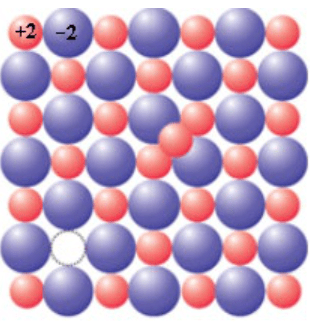(b) Frenkel defect

Red ball indicate $\mathrm{Zn}^{+2}$ ion

Blue ball indicate $S^{-2}$ ion

(ii) AgBr shows both Frenkel defect and Schottky defect.

AgBr is an ionic crystal having $\mathrm{Ag}^{+} \mathrm{Br}^{-}$. When an equal number of cations and anions are missing from their lattice, it creates a Schottky defect.

This type of defect is shown by highly ionic compounds which have high coordination number and a small difference in the sizes of cations and anions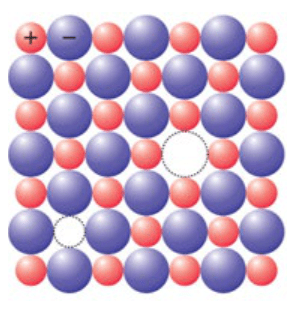(a) Schottky defect

Red ball indicate $\mathrm{Ag}^{+}$ion

Blue ball indicate $\mathrm{Br}^{-}$ion

Frenkel defect: This type of defect is present in ionic compounds which have low coordination number larger difference in the size of cation andanions, and compounds having highly polarising cation and easily polarisable anion.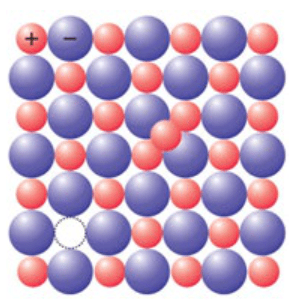(b) Frenkel defect

Red ball indicates $\mathrm{Ag}^{+}$ion

Blue ball indicates $\mathrm{Br}^{-}$ion

Question 21. Explain how vacancies are introduced in an ionic solid when a cation of higher valence is added as an impurity in it.

Solution. Let us take an example of $\mathrm{NaCl}$ doped with $\mathrm{SrCl}$ impurity. When $\mathrm{SrCl}_{2}$ is added to solid $\mathrm{NaCl}$ as an impurity, two $\mathrm{Na}^{+}$ions will be replaced and one of the sites will be occupied by $\mathrm{Sr}^{2-}$ while the other will remain vacant. Thus, we can say that when a cation of higher valance is added as an impurity to an ionic solid, two or more cations of lower valency are replaced by a cation of higher valence to maintain electrical neutrality. Therefore, some cationic vacancies are created.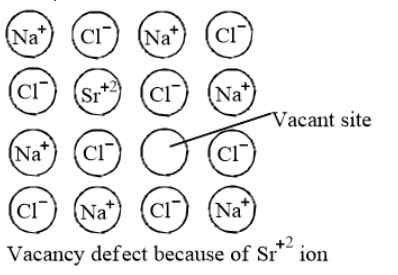Question 22. Ionic solids, which have anionic vacancies due to metal excess defect, develop colour. Explain with the help of a suitable example.

Solution. Taking the example of $\mathrm{NaCl}$, when $\mathrm{NaCl}$ is heated in an atmosphere of sodium vapours, the sodium atoms get deposited on the surface of the crystal and the chloride ions from the crystal diffuse to the surface to form $\mathrm{NaCl}$ with the deposited Na atoms. During this process, the $\mathrm{Na}$ atoms on the surface lose electrons to form $\mathrm{Na}^{+}$ions and the released electrons diffuse into the crystal to occupy the vacant anionic sites. These electrons get excited by absorbing energy from the visible light and impart yellow colour to the crystals. The colour develops because of the presence of electrons in the anionic sites. These electrons absorb energy from the visible part of radiation and get excited.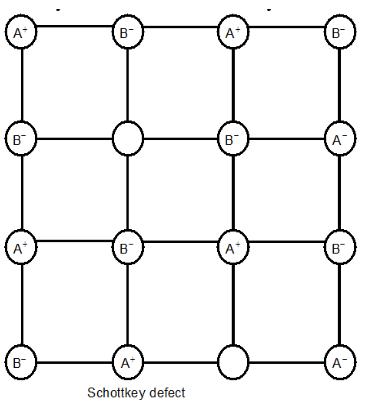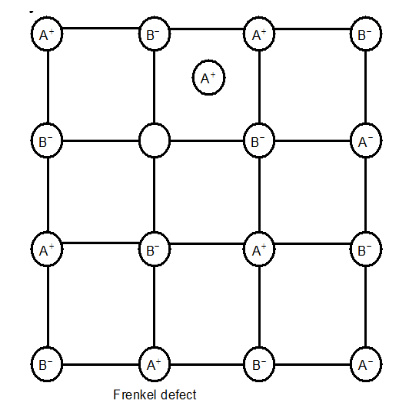Question 23. A group 14 element is to be converted into $\mathrm{n}-$ type semiconductor by doping it with a suitable impurity. To which group should this impurity belong?

Solution: The electrical conductivity of silicon or germanium can be increased by doping with some group-13 element such as $\mathrm{B}, \mathrm{Al}$ or Ga. Group-13 elements have only three valence electrons. They combine with group-14 elements to form an electron deficient bond or electron vacancy or a hole. These holes can move through the crystal like a positive charge giving rise to electrical conductivity. Since the electrical conductivity is increased because of negatively charged particles (electron), thus this is known as $\mathrm{n}$-type semiconductor.

Question 24. What type of substances would make better permanent magnets, ferromagnetic or ferrimagnetic. Justify your answer.

Solution: Ferromagnetism: When substances show permanent magnetism even in the absence of the magnetic field, this phenomenon is called as Ferromagnetism and such substances are called as Ferromagnetic substances e.g. $\mathrm{Fe}, \mathrm{Ni}, \mathrm{Co}$ and $\mathrm{CrO}_{2}$. This type of magnetism arises due to the spontaneous alignment of magnetic moments due to an unpaired electron in the same direction.

Ferrimagnetism : Substances which are expected to possess large magnetism on the basis of unpaired electrons, but actually have small magnetic momentum are called ferrimagnetic substances eg. $\mathrm{Fe}_{3} \mathrm{O}_{4}$, ferrites of the formula $\mathrm{M}^{2+}$ where $\mathrm{M}=$ $\mathrm{Mg}, \mathrm{Cu}, \mathrm{Zn}$ etc. Ferrimagnetism arises due to the unequal moments in the opposite direction resulting same net magnetic moment. On heating, these substances lose their magnetism and convert into a paramagnetic substance.

Therefore, ferromagnetic substance woul make a better permanent magnet than ferrimagnetic substance.

Question 1. Define the term ‘amorphous’. Give a few examples of amorphous solids.

Solution. Amorphous solids are the solids in which the constituent particles are of irregular shape and have short range order. They are Isotropic i.e. have the same value in all directions because they have a random arrangement of constituent particles.

Some examples of amorphous solids are glass, rubber, and plastic.

Question 2. What makes a glass different from a solid such as quartz? Under what conditions could quartz be converted into glass?

Solution. Glass is an amorphous solid in which the constituent particles have short-range order, and they are Isotropic, i.e. Same value in all directions. They are Isotropic i.e. have the same value in all directions because they have a random arrangement of constituent particles. The arrangement of the constituent particles distinguishes glass from quartz. By heating and then cooling it rapidly, quartz can be transformed into glass.

Question 3. Classify each of the following solids as ionic, metallic, molecular, network (covalent) or amorphous.

(i) Tetra phosphorus decoxide $\left(\mathrm{P}_{4} \mathrm{O}_{10}\right)$

(ii) Ammonium phosphate $\left(\mathrm{NH}_{4}\right)_{3} \mathrm{PO}_{4}$

(iii) SiC

(iv) $\mathrm{I}_{2}$

(v) $\mathrm{P}_{4}$

(vi) Plastic

(vii) Graphite

(viii) Brass

(ix) Rb

(x) LiBr

(xi) Si

Solution: Ionic $\rightarrow$ (ii) Ammonium phosphate $\left(\mathrm{NH}_{4}\right)_{3} \mathrm{PO}_{4},(\mathrm{x}) \mathrm{LiBr}$ are ionic. Ionic solid has positively charged cations and negatively charged anions. When an ionic solid is dissolved in water, the cations and the anions separate and they become free to move about in the water allowing the solution to conduct electrical current.

Metallic (viii) brass, (ix) Rb. Brass is an alloy of copper and zinc. The atoms are joined together by metallic bonds. These bonds are like huge molecular orbitals that span across the whole solid. This means the electrons in metallic solids are delocalized.

Molecular $\rightarrow$ (i) Tetra phosphorus decoxide $\left(\mathrm{P}_{4} \mathrm{O}_{10}\right)$, (iv) $\mathrm{I}_{2}$, (v) $\mathrm{P}_{4}$. Constituent particles in a molecular solid are molecules, and they are bonded with Dispersion, Dipole-dipole interaction (van der wall interaction),

Covalent (network $\rightarrow$ (iii) SiC, (vii) Graphite, (xi) Si. Constituent particles in covalent solid are ions, and they are bonded with Coulombic (electrostatics interaction ) and infinite range force of attraction. They also have high bond energy.

Amorphous $\rightarrow$ (vi) Plastic Amorphous solids are those solids in which the constituent particles are of irregular shape and have short range order. They are Isotropic i.e. Same value in all directions.

Question 4. (i) What is meant by the term coordination number?

(ii) What is the coordination number of atoms:

(a) in a cubic close-packed structure?

(b) in a body-centred cubic structure?

Solution. (i) Coordination number is defined as the number of nearest neighbours of any constituent particles present in the crystal lattice.

(ii) The coordination number of atoms:

(a) In a cubic close-packed structure is 12.

(b) In a body-centred cubic structure is 8.

Question 5. How can you determine the atomic mass of an unknown metal if you know its density and the dimension of its unit cell? Explain.

Solution: Density $=$ mass $/$ volume.

Absolute mass of atom $=$ relative atomic mass $\times 1.67 \times 10^{-24}$

Let ' $a$ ' be the edge length of a unit cell Of a crystal, 'd' be the density of the metal, 'm be the atomic mass of the metal and ' $\mathrm{N}^{\prime}$ be the number of atoms in the unit cell.

Now, density of the unit cell -Mass of the unit cell/Volume of the unit cell

$\mathrm{d}=$ number of atoms in the unit cell $\times$ relative atomic mass $\times 1.67 \times 10^{-24} /$ volume of crystal unit cell

$\mathrm{N}_{\mathrm{A}}=1 /\left(1.67 \times 10^{-24}\right)(\mathrm{amu})=6.023 \times 10^{23}$

$\mathrm{d}=\frac{\text { number of atoms in the unit cell } \times \text { relative atomic mass }(\mathrm{gm})}{6.023 \times 10^{23} \times \text { volume of crystal unit cell }}$

$\mathrm{d}=\frac{\mathrm{N} \cdot \mathrm{M}}{\mathrm{a}^{3} \mathrm{~N}_{\mathrm{A}}}$

Therefore, $M=\frac{d \cdot a^{3} N_{A}}{N}$

If the edge lengths are different (say a, b, c), the equation becomes:

$\mathrm{M}=\frac{\mathrm{d}(\mathrm{abc}) \mathrm{N}_{\mathrm{A}}}{\mathrm{N}}$

Therefore, from the above equations, we can determine the atomic mass of the unknown metal.

Question 6. ‘Stability of a crystal is reflected in the magnitude of its melting points’. Comment. Collect melting points of solid water, ethyl alcohol, diethyl ether and methane from a data book. What can you say about the intermolecular forces between these molecules?

Solution. Higher the melting point, greater is the force of attraction, and hence the stability. A substance with a higher melting point is more stable than a substance with a lower melting point. The melting points of the given substances are:

Solid water $\rightarrow 273 \mathrm{~K}=0^{\circ}$ degree centigrade, the intermolecular forces between these molecules are hydrogen bonding

Ethyl alcohol $\rightarrow 158.8 \mathrm{~K}$, the intermolecular forces between these molecules are hydrogen bonding

Diethyl ether $\rightarrow 156.85 \mathrm{~K}$, the intermolecular forces between these molecules are dipole-dipole

Methane $\rightarrow 89.34 \mathrm{~K}$, the intermolecular forces between these molecules van der Wall

From the values of the melting points of the given substances, we can observe that the intermolecular force in solid water is the strongest and that in methane is the weakest.

Question 7. How will you distinguish between the following pairs of terms:

(i) Hexagonal close-packing and cubic close-packing?

(ii) Crystal lattice and unit cell?

(iii) Tetrahedral void and octahedral void?

Solution. (i) $\quad$ HEXAGONAL CLOSE PACKING(HCP )

If spheres of IIIrd layer are placed in voids of $\mathrm{II}^{\text {nd }}$ layer which are lying exactly above the spheres of $\mathrm{I}^{\text {st }}$ layer i.e., voids of type 'a' then $\mathrm{It}$ layer and III $^{\text {rd }}$ layer will become exactly identical and this generates ABABAB pattern or the hexagonal close packing (HCP)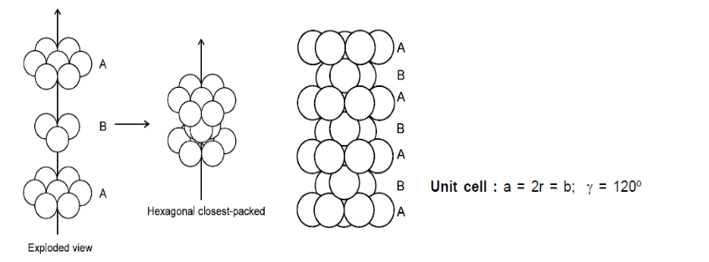(ii) CUBIC CLOSE PACKING (CCP):

If the third layer spheres are placed in those voids of IInd layer under which there are voids of the first layer of spheres (voids of type ' $b$ '), then the first and the third layer of spheres will not be identical. Such an arrangement will lead to an $\mathrm{ABC}-\mathrm{ABC}$ type of arrangement.It is also known as the cubical close packing or also as the Face Centred Cubic structure (FCC).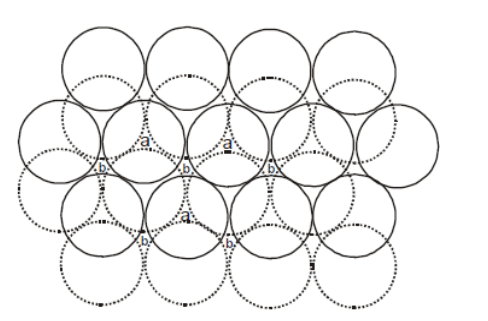In the $A B C-A B C$ Pattem the spheres of $4^{\text {th }}$ layer are vertically above the spheres of Ist layer then these consecutive layers are different from each

other, fourth layer will be idential to first layer so it will be called $\mathrm{ABC}-$ ABC repeat pattern.It is also called the ccp (cubical close packing) because a cubical type of unit cell is used for the study of this arrangement.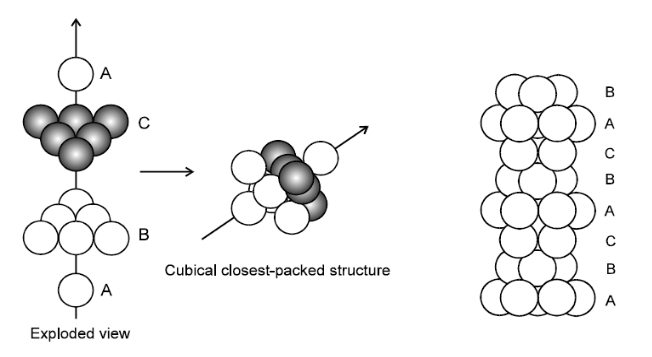(i) Orderly three-dimensional arrangements of atoms is called crystal lattice. It is a diagrammatic representation of constituent particles. Whereas, the smallest unit of a crystal lattice is called a unit cell. Repeated unit cells form a crystal lattice.

(ii)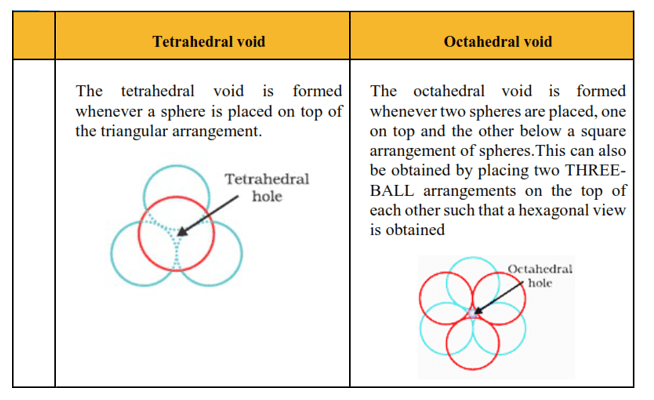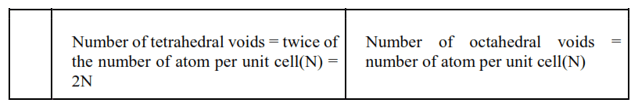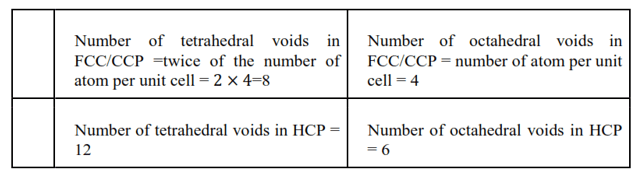Question 8. How many lattice points are there in one unit cell of each of the following lattice?

(i) Face-centred cubic

(ii) Face-centred tetragonal

(iii) Body-centred

Solution: (i) Face-centred cubic : 8 from the corners $+6$ from the faces lattice points = 14 in face-centred cubic.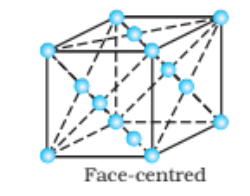(ii) Face-centred tetragonal: There are $14(8$ from the corners $+6$ from the faces) lattice points in face-centred tetragonal.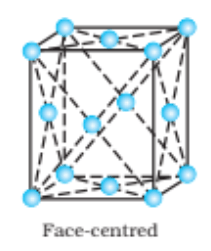(iii) Body-centred: There are 9 (1 from the centre $+8$ from the corners) lattice points in body-centred cubic.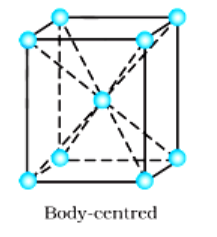Question 9. Explain

(i) The basis of similarities and differences between metallic and ionic crystals.

(ii) Ionic solids are hard and brittle.

Solution: (i) Similarities: Both in metallic and ionic crystals, atoms are held by the electrostatic force of attraction. In metallic crystals, the electrostatic force acts between opposite-charge like positive ions and the electrons. In ionic crystals, it acts between the oppositely-charged ions. Hence, metallic and ionic crystals both have high melting points.

In metallic crystals, the electrons are free to move, and so, metallic crystals can conduct electricity. Whereas, in ionic crystals, the ions are not free to move. Therefore, they cannot conduct electricity. However, in molten state or in aqueous solution, ionic crystals conduct electricity.

(ii) The constituent particles of ionic crystals are ions. These ions are held together in three - dimensional arrangement by the electrostatic force of attraction. Since the electrostatic force of attraction is very strong, the charged ions are held in fixed positions. This is the reason why ionic crystals are hard and brittle.

Question 10. Calculate the efficiency of packing in case of a metal crystal for

(i) simple cubic

(ii) body-centred cubic

(iii) face-centred cubic (with the assumptions that atoms are touching each other).

Solution. (i) Simple cubic

The particles are located only at the corners of the cube and touch each along the edge.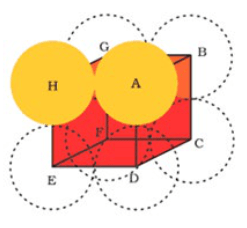Let us consider edge length of the cube to be 'a' and the radius of each particle be $\mathrm{r}$.

We can write:

$\mathrm{a}=2 \mathrm{r}$

Now, the volume of the cubic unit cell $=\mathrm{a}^{3}$

$=(2 r)^{3}$

$=8 r^{3}$

We know that the number of particles per unit cell is packing efficiency = Volume occupied by two spheres in the unit cell $* 100 /$ Total volume of the unit cell

$=\frac{\frac{4}{3} \times\left(\pi r^{3}\right) \times 100}{(8 r)^{3}}$

$=52.4 \%$

(ii) Body -centred cubic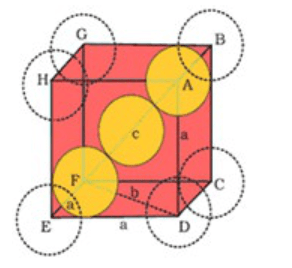From the above figure it can be observed that the atom at the centre touches the other two atoms that are diagonally arranged.

From $\Delta \mathrm{FED}$, we have:

$\mathrm{b}^{2}=2 \mathrm{a}^{2}$

$\mathrm{b}=\sqrt{2} \mathrm{a}$

Again, from $\Delta \mathrm{AFD}$, we have:

$c^{2}=a^{2}+b^{2}$

$c^{2}=a^{2}+2 a^{2}$

$c^{2}=3 a^{3}$

$\mathrm{b}=\sqrt{3} \mathrm{a}$

Let the radius of the atom be r.

Length of the body diagonal, $\mathrm{c}=4 \mathrm{r} \sqrt{3} \mathrm{a}=4 \mathrm{r}$

The volume of the cube, $a^{3}=(4 r / \sqrt{3} a)^{3}$

A body - centred cubic lattice contains total of 2 atoms.

So, the volume of the occupied cubic lattice

$=\frac{8}{3} \pi r^{3}$

Packing efficiency $=$ volume occupied by two spheres in the unit cell $* 100 /$ Total volume of the unit cell

$=\frac{\frac{8}{3} \pi r 3 \times 100}{(4 r / \sqrt{3})^{3}} \%$

$=68 \%$

(iii) Face-centred cubic

Le the edge length of the unit cell be 'a', and the length of the face diagonal AC be b.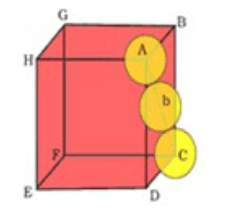From $\triangle \mathrm{ABC}$, we have

$b^{2}=a^{2}+a^{2}$

$\mathrm{b}^{2}=\mathrm{a}^{2}+\mathrm{a}^{2}$

$b^{2}=2 a^{2}$

$b=\sqrt{2} a$

Let the radius of the atom be r.

Now, from the figure, it can be observed that:

$\mathrm{b}=4 \mathrm{r}$

$\sqrt{2} \mathrm{a}=4 \mathrm{r}$

$a=2 \sqrt{2} r$

Now, the volume of the cube, $a^{3}=(2 \sqrt{2} r)^{3}$

We know that the number of atoms per unit cell in FCC is $4 .$ 16

So, the volume of the occupied unit cell $=\frac{16}{3 \pi r^{3}}$

Packing efficiency $=$ Volume occupied by two spheres in the unit cell $*$ $100 /$ Total volume of the unit cell

$=\frac{\frac{16}{3 \pi r^{3}} \times 100}{(2 / \sqrt{2})^{3}}$

$=74 \%$

Question 11. Silver crystallises in fcc lattice. If edge length of the cell is $4.07 \times 10^{-8} \mathrm{~cm}$ and density is $10.5 \mathrm{~g} \mathrm{~cm}^{-3}$, calculate the atomic mass of silver.

Solution: Given: edge length of the cell $(\mathrm{a})=4.07 \times 10^{-8} \mathrm{~cm}$

Density $(\mathrm{d})=10.5 \mathrm{~g} \mathrm{~cm}^{-3}$

We also know that: the lattice is fcc type, the number of atoms per unit cell, $\mathrm{Z}=4$

$\mathrm{N}_{\mathrm{A}}=6.022 \times 10^{23} \mathrm{~mol}^{-1}$

Using the relation:

$\mathrm{d}=\mathrm{Z} . \mathrm{M} / \mathrm{a}^{3} \mathrm{~N}_{\mathrm{A}}$

$\mathrm{d}=\frac{\mathrm{ZM}}{\mathrm{a}^{3} \mathrm{~N}_{\mathrm{A}}}$

Therefore, $M=\frac{d a^{3} N_{A}}{z}$

$=10.5 \mathrm{~g} \mathrm{~cm}^{-3} \mathrm{x}\left(4.077 \times 10^{-8} \mathrm{~cm}\right)^{3} \times 6.022 \times 10^{23} \mathrm{~mol}^{-1}$

$=107.13 \mathrm{~g} \mathrm{~mol}^{-1}$

Therefore, the atomic mass of silver $=107.13 \mathrm{u}$

Question 12. A cubic solid is made of two elements $\mathrm{P}$ and $\mathrm{Q}$. Atoms of $\mathrm{Q}$ are at the corners of the cube and $P$ at the body-centre. What is the formula of the compound? What are the coordination numbers of $\mathrm{P}$ and $\mathrm{Q}$?

Solution. Since the atoms of $Q$ are present at the corners of the cube.

The number of atoms of $Q$ in one unit cell $8 \times 1 / 8=1$

It is also mentioned that the atoms of P are present at the body-centre (inside the lattice).

Therefore, the number of atoms of $\mathrm{P}$ in one unit cell $=1$

This means that the ratio of the number of $\mathrm{P}$ atoms to the number of $\mathrm{Q}$ atoms, $\mathrm{P}: \mathrm{Q}=$ $1: 1$

Hence, the formula of the compound is PQ.

The coordination number of both $\mathrm{P}$ and $\mathrm{Q}$ is 8 .

Question 13. Niobium crystallises in a body-centred cubic structure. If the density is $8.55 \mathrm{~g} \mathrm{~cm}^{-3}$, calculate the atomic radius of niobium using its atomic mass $93 \mathrm{u}$.

Solution. Given: The density of niobium $(\mathrm{d})=8.55 \mathrm{~g} \mathrm{~cm}^{-3}$

The atomic mass of niobium $(\mathrm{M})=93 \mathrm{~g} \mathrm{~mol}^{-1}$

We also know that: As the lattice is bec type, the number of atoms per unit cell, $\mathrm{Z}=$ 2

$\mathrm{N}_{\mathrm{A}}=6.022 \times 10^{23} \mathrm{~mol}^{-1}$

Applying the relation:

$\mathrm{d}=\frac{\mathrm{ZM}}{\mathrm{a}^{3} \mathrm{~N}_{\mathrm{A}}}$

Therefore,

$\mathrm{a}^{3}=\left(2 \times 93 \mathrm{~g} \mathrm{~mol}^{-1}\right) /\left(8.5 \mathrm{~g} \mathrm{~cm}^{-3} \times 6.022 \times 10^{23} \mathrm{~mol}^{-1}\right)$

$\mathrm{a}^{3}=3.612 \times 10^{-23} \mathrm{~cm}^{3}$

So, $\mathrm{a}=3.306 \times 10^{-8} \mathrm{~cm}$

For body -centred cubic unit cell:

$r=\sqrt{3} / 4 a$

$=1.432 \times 10^{-8} \mathrm{~cm}$

$=1432 \times 10^{-9} \mathrm{~cm}$

Question 14. If the radius of the octahedral void is r and radius of the atoms in close packing is R, derive relation between r and R.

Solution.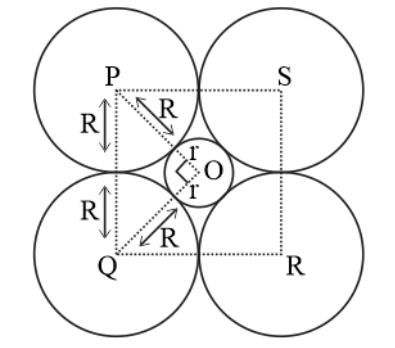A sphere with centre 0 is fitted into the octahedral void as shown in the figure. It can be seen from the figure that $\Delta \mathrm{POQ}$ is right-angled.

$\angle \mathrm{POQ}=90^{\circ}$

Now applying Pythagoras theorem, we can write,

$\mathrm{PQ}^{2}=\mathrm{PO}^{2}+\mathrm{OQ}^{2}$

$(2 R)^{2}=(R+r)^{2}+(R+r)^{2}$

$(2 R)^{2}=\sqrt{2}(R+r)^{2}$

$\sqrt{2} \mathrm{R}=\mathrm{R}+\mathrm{r}$

$r=\sqrt{2 R}-R$

$\mathrm{r}=0.414 \mathrm{R}$

Question 15. Copper crystallises into FCC lattice with edge length $3.61 \times 10^{-8} \mathrm{~cm}$. Show that the calculated density is in agreement with its measured value of $8.92 \mathrm{~g} \mathrm{~cm}^{-3}$.

Solution. $\mathrm{a}=3.61 \times 10^{-8} \mathrm{~cm}$

The lattice is of fcc type. Therefore, the number of atoms per unit cell, $Z=4$

Atomic mass, $\mathrm{M}=63.5 \mathrm{~g} \mathrm{~mol}^{-1}$

We also know that, $\mathrm{N}_{\mathrm{A}}=6.022 \times 10^{23} \mathrm{~mol}^{-1}$

Applying the relation:

$\mathrm{d}=\frac{\mathrm{ZM}}{\mathrm{a}^{3} \mathrm{~N}_{\mathrm{A}}}$

$=\left(4 \times 63.5 \mathrm{~g} \mathrm{~mol}^{-1}\right) /\left(3.61 \times 10^{-8} \mathrm{~cm} \times 6.022 \times 10^{23} \mathrm{~mol}^{-1}\right)$

$=8.97 \mathrm{~g} \mathrm{~cm}^{-3}$

The measured value of density given is $8.92 \mathrm{~g} \mathrm{~cm}^{-3}$. Hence, the calculated density $8.97 \mathrm{~g} \mathrm{~cm}^{-3}$ is in agreement with its measured value.

Question 16. Analysis shows that nickel oxide has the formula $\mathrm{Ni}_{0.98} \mathrm{O}_{1.00}$. What fractions of nickel exist as $\mathrm{Ni}^{2+}$ and $\mathrm{Ni}^{3+}$ ions?

Solution. Nickel oxide is $\mathrm{Ni}_{0.98} \mathrm{O}_{1.00}$.

The ratio of the number of $\mathrm{Ni}$ atoms to the number of 0 atoms,

Ni: $0=0.98: 1.00=98: 100$

Now total charge on $1000^{2-}$ ions $=100 \times(-2)$

$=-200$

Let the charge of $\mathrm{Ni}^{2+}$ ions be $\mathrm{x}$.

So, the charge of $\mathrm{Ni}^{3+}$ ions is $(98-\mathrm{x})$

Now, total charge on $\mathrm{Ni}^{2+}$ ions $=\mathrm{x}(+2)=+2 \mathrm{x}$

And, total charge on $\mathrm{Ni}^{3+}$ ions $=(98-\mathrm{x})(+3)$

$=294-3 x$

Since, the compound is neutral,so by charge and atom conservation law we can write:

$2 x+(294-3 x)+(-200)=0$

$\Rightarrow-x+94=0$

$\Rightarrow \mathrm{x}=94$.

Therefore, the number of $\mathrm{Ni}^{2+}$ ions $=94$

And, the number of $\mathrm{Ni}^{3+}$ ions $=98-94=4$

Hence, the fraction of nickel that exists as $\mathrm{Ni}^{2+}=94 / 98=0.959$

And, the fraction of nickel that exists as $\mathrm{Ni}^{3+}=4 / 98=0.041$

Question 17. What is a semiconductor? Describe the two main types of semiconductors and contrast their conduction mechanism.

Solution. Substances whose conductance lies between $10^{-6}$ to $10^{4} \mathrm{ohm}^{-1} \mathrm{~m}^{-1}$ are called semiconductors.

There are two types of semiconductors. They are:

(i) n -type semiconductor

(ii) $\mathrm{p}-$ type semiconductor

(i) $\mathrm{n}$-type semiconductor: The semiconductor whose increased conductivity is a result of negatively-charged electrons is called an n -type semiconductor. When a crystal of a group 14 element such as $\mathrm{Si}$ or Ge is doped with an element of group 15 such as $\mathrm{P}$ or As, an $\mathrm{n}$-type semiconductor is formed.

Si and Ge have four valence electrons each. In their crystals, each atom forms four covalent bonds. On the Other hand, $\mathrm{P}$ and As contain five valence electrons each. When Si or Ge is doped with $\mathrm{P}$ or AS, the latter occupies some of the lattice Sites in the crystal. Four out of five electrons are used in the formation of four covalent bonds with four neighbouring Si or Ge atoms. The remaining fifth electron becomes delocalised and increases the conductivity of the doped $S i$ or Ge.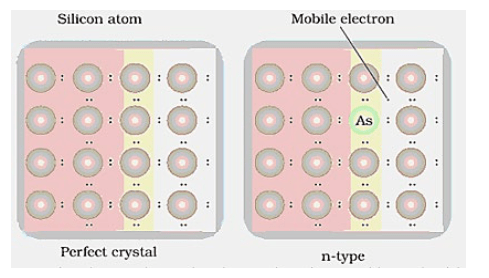p-type semiconductor: The semiconductor whose increased in conductivity is a result of electron-hole is called a p -type semiconductor. When a crystal of a group 14 element such as Si or Ge is doped with a group 13 element such as $\mathrm{B}, \mathrm{Al}$, or $\mathrm{Ga}$ (which contains only three valence electrons), a p-type of semiconductor is generated.

When a crystal of $\mathrm{Si}$ is doped with $\mathrm{B}$, the three electrons used in the formation of three covalent bonds and an electron-hole is created. An electron from the neighbouring atom can come and fill this electron hole, but in doing so, it would leave an electron-hole at its original position. The process appears as if the electron-hole has moved in a direction opposite to that of the electron that filled it. Thus, when an electric field is applied, electrons will move toward the positively-charged plate through electron holes. However, it will appear as if the electron holes are positively-charged and are moving toward the negatively- charged plate.

Question 18. Non-stoichiometric cuprous oxide, $\mathrm{Cu}_{2} \mathrm{O}$ can be prepared in laboratory. In this oxide, copper to oxygen ratio is slightly less than $2: 1$. Can you account for the fact that this substance is a $\mathrm{p}$-type semiconductor?

Solution. $\mathrm{Cu}_{2} \mathrm{O}$ prepared in the laboratory, copper to oxygen ratio is slightly less than $2: 1 .$ This means that the number of $\mathrm{Cu}_{2}^{+2}$ ions are slightly less than the number of $\mathrm{O}^{2-}$ ions. This is due to the reason that some $\mathrm{Cu}_{2}^{+2}$ ions are replaced by $\mathrm{Cu}^{2+}$ ions thereby creating holes. As a result, the substance conducts electricity with the help of these positive holes. Hence, the substance is a p-type semiconductor.

Question 19. Ferric oxide crystallises in a hexagonal close-packed array of oxide ions with two out of every three octahedral holes occupied by ferric ions. Derive the formula of the ferric oxide.

Solution. Let the number of oxide $\left(0^{2-}\right)$ ions be $x$.

So, the number of octahedral voids $=x$

It is mentioned that two out of every three octahedral holes are occupied by ferric ions.

So, number of ferric $\left(\mathrm{Fe}^{3+}\right)$ ions $=2 \mathrm{x} / 3$

Therefore, the ratio of the number of $\mathrm{Fe} 3+$ ions to the number of $\mathrm{O}^{2-}$ ions,

$\mathrm{Fe}^{3+}: 0^{2-}=2 / 3 \mathrm{x}: \mathrm{x}$

$=2 / 3: 1$

$=2: 3$

Hence, formula of the ferric oxide is $\mathrm{Fe}_{2} \mathrm{O}_{3}$.

Question 20. Classify each of the following as being either a p-type or an n-type semiconductor:

(i) Ge doped with In

(ii) Si doped with $\mathrm{B}$.

Solution. Electrical conductivity of germanium can be increased by doping with group-13 elements B, Al, In Ga, Tl. Group-13 elements have only three valence electrons. They combine with the group-14 element $(\mathrm{Si}, \mathrm{Ge}, \mathrm{Sn}, \mathrm{Pb})$ to form an electron deficient bond or electron vacancy or a hole. These holes can move through the crystal like a positive charge giving rise to electrical conductivity.

Group-14 (Si) elements doped with group-13 (B) elements,they behave as p-type semiconductors. In the presence of electric field, the holes move in a direction opposite to that of electrons.

when Ge doped with In and Si doped with B is p −type semiconductor

Question 21. Gold (atomic radius $=0.144 \mathrm{~nm}$ ) crystallises in a face-centred unit cell. What is the length of a side of the cell?

Solution. Given that

Radius of atom $(\mathrm{r})=0.144 \mathrm{~nm}$

We know that: For a face-centred unit cell relation between edge length is

edge length $(\mathrm{a})=2 \sqrt{2}$ radius of an atom

edge length $(a)=2 \sqrt{2} r$

Put the values in we get

Edge $(a)=2 \times 1.414 \times 0.144 \mathrm{~nm}$

Edge $(a)=0.407 \mathrm{~nm}$

The length of a side of the unit cell $=0.407 \mathrm{~nm}$

Question 22. In terms of band theory, what is the difference

(i) between a conductor and an insulator

(ii) between a conductor and a semiconductor?

Solution. (i) The valence band of a conductor is partially-filled or it overlaps with a higher energy, unoccupied conduction band.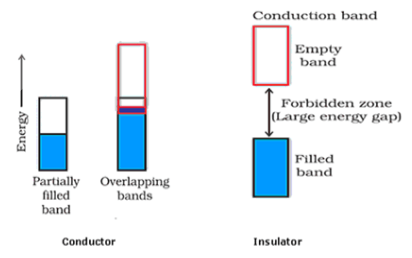In the case of an insulator, the valence band is fully-filled and there is a large gap between the valence band and the conduction band.

(ii) In the case of a conductor, the valence band is partially-filled or it overlaps with a higher energy, unoccupied conduction band. So, the electrons can flow easily under an applied electric field.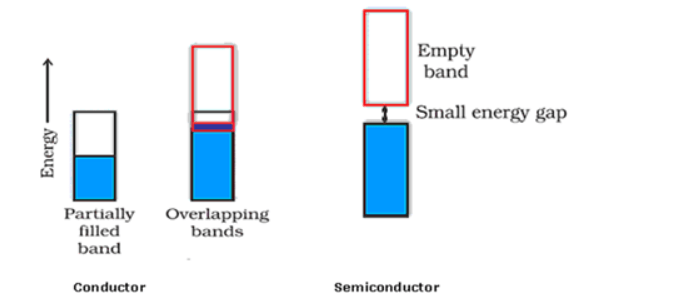Whereas, the valence band of a semiconductor is filled and there is a small gap between the valence band and the next higher conduction band. Hence, some electrons can jump from the valence band to the conduction band and conduct electricity.

Question 23. Explain the following terms with suitable examples:

(i) Schottky defect

(ii) Frenkel defect

(iii) Interstitials and

(iv) F −centres.

Solution: (i) Schottky defect:

If in an ionic crystal of the type $A+B-$, an equal number of cations and anions are missing from their lattice. It is called a Schottky defect.

This type of defect is shown by highly ionic compounds which have

(i) High Coordination number and

(ii) A small difference in the sizes of cations and anions

A few examples of ionic compounds exhibiting Schottky defect are $\mathrm{NaCl}, \mathrm{KCl}, \mathrm{KBr}$ and $\mathrm{CsCl}$.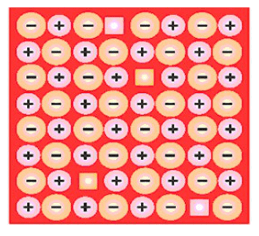(ii) Frenkel defect: When an ion is missing from its correct lattice sites (causing a vacancy or a hole) and occupies an interstitial site, electrical neutrality, as well as stoichiometry of the compounds, are maintained. This type of defect is called a Frenkel defect. Since cations are usually smaller, it is more common to find the cations occupying interstitial sites.

This type of defect is present in ionic compounds which have

(i) Low co ordinations number

(ii) Larger difference in the size of cation and anions

(iii) Compounds having highly polarising cation and easily polarisable anion. A few examples of ionic compounds exhibiting this defect are $\mathrm{AgCl}, \mathrm{AgBr}, \mathrm{AgI}, \mathrm{ZnS}$ etc. Consequences of Frenkel defect

(a) As no ions are missing from the crystal lattice as a whole, therefore,the density of the solid remains the same

(b) The closeness of like charges tends to increases the dielectric constant of the crystal

(c) The crystal conducts electricity to a small extent by an ionic mechanism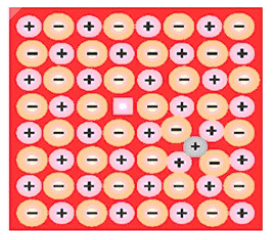(iv) Interstitials: Interstitials or Interstitial defect is shown by non-ionic solids. This type of defect is created when some constituent particles (atoms or molecules) occupy an interstitial site of the crystal. The density of a substance increases because of this defect.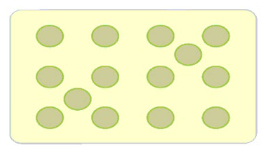(v) $\mathrm{F}$ - centres: Metal excess or cation excess defect:- If no. of missing anion is more than number of missing cations. - To maintain electrical neutrality, some electrons are trapped at anionic vacancies. Because of these extra electrons the electrical and optical (colour) properties of the compound get modified. So these locations are also known as colour centres $(\mathrm{F}-$ centres).

$\mathrm{ZnO}$ is white in colour at room temperature. On heating some oxygen ions get released in the form of $\mathrm{O}_{2}$ and are trapped at their locations. Because of this it becomes yellow in color.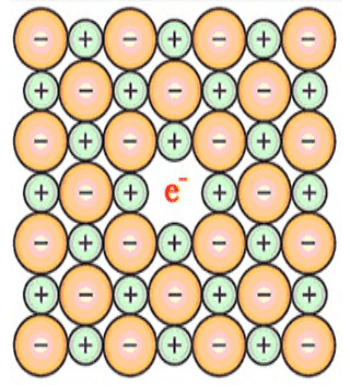Question 24. Aluminium crystallises in a cubic close-packed structure. Its metallic radius is 125 pm.

(i) What is the length of the side of the unit cell?

(ii) How many unit cells are there in $1.00 \mathrm{~cm}^{3}$ of aluminium?

Solution: (i) For cubic close-packed structure:

$\mathrm{a}=2 \sqrt{2} \mathrm{r}$

$\mathrm{a}=2 \sqrt{2} 125$

$=353.55 \mathrm{pm}$

(ii) Volume of one unit cell $=(353.55 \mathrm{pm})^{3}$

$=4.419 \times 10^{7} \mathrm{pm}^{3}$

$=4.4 \times 10^{7} \times 10^{-30} \mathrm{~cm}^{3}$

$=4.4 \times 10^{-23} \mathrm{~cm}^{3}$

Therefore, the number of unit cells in

$1.00 \mathrm{~cm}^{3}$ of $\mathrm{Al}=1.00 \mathrm{~cm}^{3} /\left(4.4 \times 10^{-23} \mathrm{~cm}^{3}\right)$

$=2.27 \times 10^{22}$

Question 25. If $\mathrm{NaCl}$ is doped with $10^{-3}$ mol $\%$ of $\operatorname{SrCl}_{2}$, what is the concentration of cation vacancies?

Solution. It is given that $\mathrm{NaCl}$ is doped with $10^{-3} \mathrm{~mol} \%$ of $\mathrm{SrCl}_{2}$. This signifies that $100 \mathrm{~mol}$ of $\mathrm{NaCl}$ is doped with $10^{-3} \mathrm{~mol}$ of $\mathrm{SrCl}_{2}$. Hence, $1 \mathrm{~mol}$ of $\mathrm{NaCl}$ is doped with $10^{-3} / 100 \mathrm{~mol}$ of $\mathrm{SrCl}_{2} .=10^{-5} \mathrm{~mol}$ of $\mathrm{SrCl}_{2}$ by removing of $2 \mathrm{Na}^{+}$ions. One cation vacancy is produced by one $\mathrm{Sr}^{2+}$ ion.

The concentration of the cation vacancies produced by $10^{-5} \mathrm{~mol}$ of $\mathrm{Sr}^{2+}$ ions $=$ $10^{-5} \times 6.022 \times 10^{23}=6.022 \times 10^{18} \mathrm{~mol}^{-1}$

Hence, the concentration of cation vacancies created by $\operatorname{SrCl}_{2}$ is $6.022 \times 10^{18}$ per mol of $\mathrm{NaCl}$

Question 26. Explain the following with suitable examples:

(i) Ferromagnetism

(ii) Paramagnetism

(iii) Ferrimagnetism

(iv) Antiferromagnetism

(v) 12 − 16 and 13 − 15 group compounds.

Solution: (i) Ferromagnetism: When substances show permanent magnetism even in the absence of the magnetic field, this phenomenon is called as Ferromagnetism and such substances are called as Ferromagnetic substances e.g. Fe, Ni, Co and $\mathrm{CrO}_{2}$. This type of magnetism arises due to the spontaneous alignment of magnetic moments due to an unpaired electron in the same direction.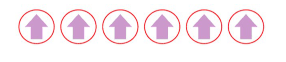Schematic alignment of magnetic moments in ferromagnetic substances

(ii) Paramagnetism: When substances get attracted by the external magnetic field, they are called paramagnetic substances, and the phenomenon is called paramagnetism. Atoms, ion or molecules containing unpaired electrons show this property, eg. $\mathrm{O}_{2} \mathrm{Cu}^{2+}, \mathrm{Fe}^{3+}$ etc. these substances lost their magnetism in the absence of a magnetic field.

(iii) Ferrimagnetism: Substances which are expected to possess large magnetism on the basis of unpaired electrons, but actually have small magnetic momentum are called ferrimagnetic substances eg. $\mathrm{Fe}_{3} \mathrm{O}_{4}$, ferrites of the formula $\mathrm{M}^{2+}$ where $\mathrm{M}=\mathrm{Mg}, \mathrm{Cu}, \mathrm{Zn}$ etc. Ferrimagnetism arises due to the unequal moments in the opposite direction resulting same net magnetic moment. On heating, these substances lose their magnetism and convert into a paramagnetic substance.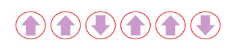Schematic alignment of magnetic moments in ferrimagnetic substances

(iv) Antiferromagnetism: Substances that possess paramagnetism or ferromagnetism on the basis of unpaired electrons but they possess zero net magnetic moment are called antiferromatic substances and the phenomenon is known as Anti-ferromagnetism.

For example: MnO. Anti-ferromagnetism is due to the presence equal number of magnetic moments in the opposite direction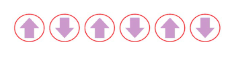Schematic alignment of magnetic moments in antiferromagnetic substances (v) $12-16$ and $13-15$ group compounds: The $12-16$ group compounds are prepared by combining group 12 and group 16 elements and the $13-15$ group compounds are prepared by combining group 13 and group 15 elements. These compounds are prepared to stimulate average valence of four as in Ge or Si.

Example of some compounds belonging to $12-16$ group are $\mathrm{ZnS}, \mathrm{Cds}$.

and $13-15$ group are InSb, GaAs.